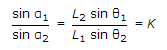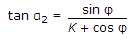# Civil Engineering - Docks and Harbours - Discussion

### Discussion :: Docks and Harbours - Section 1 (Q.No.34)

34.

The horizontal angles from the boat between A and B and B and C, the stations on the shore are respectively θ1 and θ2. The distances AB = L1 and BC = L2. For calculating the angle α2 at C between the boat and station B is obtained by using the following formula: where (β is horizontal angle between A and C at B).

 [A].[B]. αt tan α2 = 360° - (θ1 + θ2 + β) = φ [C].[D]. all the above.

Explanation:

No answer description available for this question.